Raking a survey sample, tutorial in Excel

2017-10-20

This tutorial shows how to rake the results of a survey by generating weights that make the surveyed sample more representative of the population.

Dataset for raking a survey

An Excel sheet with both the data and the results can be downloaded by clicking here.

The data correspond to a survey based on 200 employees of a company on their satisfaction at work (simulated data). Two auxiliary variables were included in the survey: gender (1: male, 2: female) and age (1:45). The proportions of these variables in the entire company are known (marginal control totals). There are 10000 employees in this company.

In the Sat column, you can find a satisfaction score that won’t be used in this step of the analysis. We are interested in finding raking weights that can be applied to our survey sample in order to obtain similar proportions for the modalities of the auxiliary variables, in the survey sample and in the population (Deming and Stephan, 1940).

Setting up the raking of a survey

After opening XLSTAT, click the Preparing data button in the ribbon and select Raking survey (see below).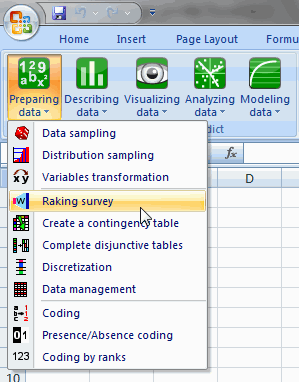Once you've clicked on the button, the dialog box appears. Select the data on the Excel sheet. You only need to select the auxiliary variables (gender and age).

The marginal control totals have to be selected all together in the same order as the data to be raked on (one column for each variable; one row for each modality).

Each column has to sum to the same value (here 10000). As we selected the column title for the variables, we left the option Variable labels activated.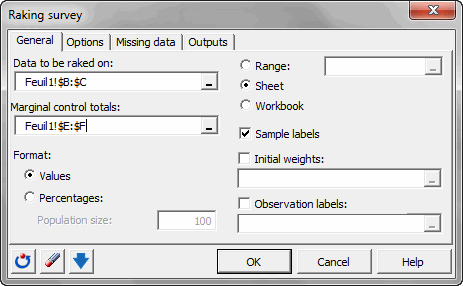In the Options tab, we select Raking Ratio as estimation method.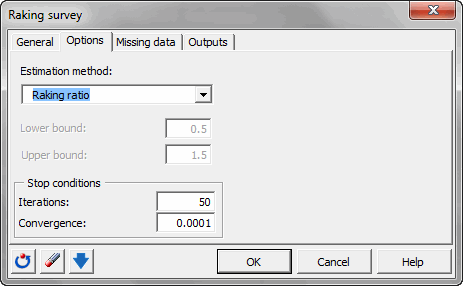Once you have clicked on the OK button, the computation starts. The results will then be displayed.

Interpreting the results of the raking of a survey

The first results displayed by XLSTAT are the basic statistics associated with the auxiliary variables before raking.

Then, the second table contains the final weights for each observation, the initial auxiliary variables and the weights ratios (final weights / initial weights) (see below for observations 1 to 13).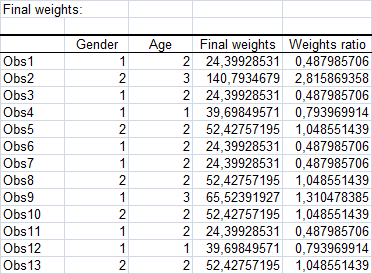Then, the basic statistics after raking are displayed. We can see that using the obtained weights, the statistics are equal in the survey sample and in the population.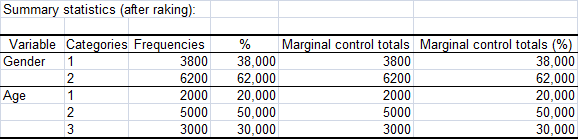We have obtained final weights that are adapted and that can be used for further analysis of the employees' satisfaction.

Contact our technical support team: support@xlstat.com

https://cdn.desk.com/
false
desk